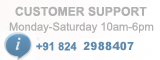# PIC Development Board

/*
PIC Development Board
(c) Researchdesignlab.com
* Test configuration:
MCU: PIC16F877A
Dev.Board: PIC
Oscillator: 20.0 MHz
Software: MPLAB IDE v8.92(HI-TECH_C)
*/

#include <htc.h>
#include <stdio.h> // Define I/O functions
#define XTAL 20000000
#define BAUD_RATE 9.6 //9600 Baudrate
#define BAUD_VAL (char)(XTAL/ (16 * BAUD_RATE )) - 1; //Calculation For 9600 Baudrate @20Mhz
#define EN RC0
#define RS RC1
void ScanCol(void); //Column Scan Function
void ScanRow(void); //Row Scan Function
void DelayMs(unsigned int);
void LCD_Cmd(unsigned char);
void LCD_Init(void);
void LCD_Display( char *addr);
void LCD_SendDataByte(unsigned char);

unsigned char KeyArray= { '1','2','3','4',
'5','6','7','8',
'9','A','B','C',
'D','E','F','0'};
//Keypad value Initialization Function

unsigned char Count={0,0,0,0,0,0,0,0,0,0,0,0,0,0,0,0};
int Col=0,Row=0,count=0,i,j;

void main()
{

TRISD=0x00; //set registerD as output
TRISC=0x00; //set register C as output
LCD_Init(); //initialize LCD

DelayMs(1000);
nRBPU=0; //Enable PORTB Pullup values

while(1)
{
TRISB=0X0f; // Enable the 4 LSB as I/P & 4 MSB as O/P
PORTB=0X00;
while(PORTB==0x0f); // Get the ROW value
ScanRow();

TRISB=0Xf0; // Enable the 4 LSB as O/P & 4 MSB as I/P
PORTB=0X00;
while(PORTB==0xf0); // Get the Column value
ScanCol();

DelayMs(1000); //provide a delay of 1s
Count[Row][Col]++; // Count the Pressed key
LCD_Cmd(0X01); //clear the LCD
LCD_Cmd(0X80); //1st row of the LCD
LCD_SendDataByte(KeyArray[Row][Col]); //send keypad value and display on LCD
DelayMs(1000); //provide delay of 1s
}

}

void ScanRow() // Row Scan Function
{

switch(PORTB)
{
case 0x07:
Row=3; // 4th Row
break;
case 0x0b:
Row=2; // 3rd Row
break;
case 0x0d:
Row=1; // 2nd Row
break;
case 0x0e:
Row=0; // 1st Row
break;
}
}

void ScanCol() // Column Scan Function
{

switch(PORTB)
{
case 0x70:
Col=3; // 4th Column
break;
case 0xb0:
Col=2; // 3rd Column
break;
case 0xd0:
Col=1; // 2nd Column
break;
case 0xe0:
Col=0; // 1st Column
break;
}
}
/*LCD CODE*/

void LCD_Delay() //delay routine
{
__delay_ms(1);
}

void LCD_Cmd(unsigned char cmd) //this function is to write command to the LCD
{

PORTB=cmd;
RS=0; //Set RS pin to low in order to send a command to the LCD
EN=1; //set EN pin to high in order to send high pulse
LCD_Delay(); //give a small delay
EN=0; //set EN pin to low in order to make pulse low
LCD_Delay(); //give a small delay
}

void LCD_Init() //Initializing LCD
{
unsigned char cmd={0X38,0X06,0X0F,0X01,0X80},Count;
//0x38 represents 5x7 matrix ,0x06 represent entry mode,0x0f represent display on cursor blinking,0x01 represents clearing the LCD,0x80 represents 1st row
for(Count=0;Count<5;Count++)
LCD_Cmd(cmd[Count]);
}

void LCD_SendDataByte(unsigned char data) //this function is to write a byte on LCD
{
PORTB=data;
RS=1; //make RS pin high inorder to send a data
EN=1; //set enable pin to high in order to send high to low pulse
LCD_Delay(); //provide a small delay
EN=0;
LCD_Delay();
}
void LCD_Display( char *addr) //this function is to display a string on LCD
{